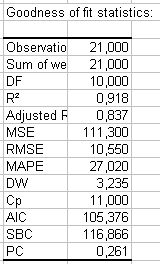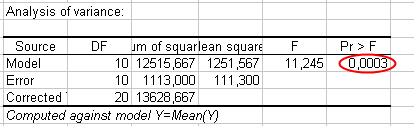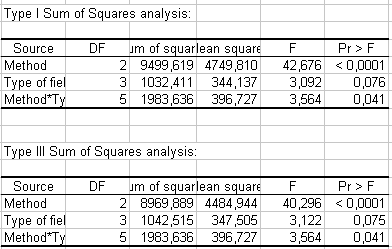# Two-way unbalanced ANOVA with interactions in Excel

2018-11-20

This tutorial will help you set up and interpret a two-way Analysis of Variance (ANOVA) on unbalanced data in Excel using the XLSTAT software.
Not sure this is the modeling feature you are looking for? Check out this guide

## Dataset for a two-way unbalanced ANOVA with interactions

An Excel sheet with both the data and the results can be downloaded by clicking on the button below:

The data correspond to an experiment in which four different methods for growing crops were tested on four different types of fields (same soil but different light exposure).

The yield was measured after the harvest. Because the 3rd method was not tested on the 4th type of field (because of a lack of seeds), and the 2nd method on the 4th type of field (because of a hail storm), the experiment is a typical example of an unbalanced ANOVA. We have performed an ANOVA with interactions in order to determine the interactions between the types of methods used and the types of fields.

With XLSTAT's ANOVA function, we can find out if the growing method has a significant effect on the yield by controlling the type of field and the interaction between the method and the type of field.

## Setting up a two-way unbalanced ANOVA with interactions

After opening XLSTAT, select the XLSTAT / Modeling data / ANOVA command/Once you've clicked on the button, the ANOVA dialog box appears.

Select the data on the Excel sheet. The Dependent variable (or variable to model) is here the "Yield".

Our aim is to determine the effect of the method, the type of field and the interaction between the two on the variability of the yield.

As we selected the column title for the variables, we left the option Variable labels activated.

In XLSTAT, it is possible to select the data in two different ways for the ANOVA. The first is in the form of columns, one column for the dependent variable, and the others for the explanatory variables.The second way of selecting the data is in the form of a table, with, in columns, the modalities of an explanatory variable, and in lines, the modalities of the second explanatory variable.The interactions option is activated on the options tab, and the maximum level of intraction is set to 2.

We left the constraint option at a1=0, meaning that we want the model to be built on the assumption that the method "1" has the standard effect on the yield.

Although you have to apply a constraint to the model in ANOVA for theoretical reasons, it will not affect the results (goodness of fit, predictions). The only difference it makes is in the actual writing of the model.In the Outputs tab, the Type SS options were activated because we want the model to take the interactions into account, and because we want to analyze the F values given in the Type I SS, and Type III SS tables (SS stands for sum of squares).The computations begin once you have clicked on the OK button. A dialog box is displayed so that you can confirm which factors have to be taken into account in the model.The results will then be displayed.

## Interpreting the results of a two-way unbalanced ANOVA with interactions

The first results displayed by XLSTAT are the goodness of fit coefficients including the R’² (coefficient of determination), and the adjusted R’².

The coefficient of determination (0.92) gives us a fair idea of the extent to which the variability of the modeled variable (the yield) can be explained by the explanatory variables (the method, the type of field, and their interaction). In this particular example, 92% of the variability is explained. The remaining 8 percent are hidden in other variables, which the model classifies as "random effects."It is important to examine the results of the analysis of variance table (see below). The results enable us to determine whether or not the explanatory variables bring significant information (null hypothesis H0) to the model. In other words, it's a way of asking yourself whether it is valid to use the mean to describe the whole population, or whether the information brought by the explanatory variables is of value or not.Given that the probability corresponding to the Fisher's F is lower than 0.0003, it means that we would be taking a 0.03% risk in assuming that the null hypothesis (no effect of the two explanatory variables and their interaction) is wrong.

Therefore, we can conclude with confidence that the two variables and their interaction do have a significant effect. We also want to find out if the two variables, and their interaction, provide the same amount of information. To do this, we have to examine the Type I SS and Type III SS tables.The Type I SS table is constructed by adding variables in the model one by one, and by evaluating the impact of each on the model sum of squares (Model SS). In consequence, in Type I SS, the order in which the variables are selected will influence the results.

The Type III SS table is computed by removing one variable of the model at a time to evaluate its impact on the quality of the model. This means that the order in which the variables are selected will not have any effect on the values in the Type III SS. The Type III SS is generally the best method to use to interpret results when an interaction is part of the model.

Note: the higher the Model SS, the lower the Residual SS, and therefore the greater the influence of the variable.

From the results displayed in the Type III SS table, we can see that the "Method" variable is the one that has the highest impact on the model.

When we look at the model parameters (see below), we can see that methods 2 and 3 have a positive impact on the yield. The "Type of field" has a low effect on the yield, but the influence of the interaction between the type of field and the method should not be overlooked (the confidence range is 95 percent, meaning there is a 5 percent risk factor).The table depicted above can be used to analyze the impact of the explanatory variables on the yield and/or to predict the average yield in a situation not yet covered by the experiment, such as the 3rd method and 4th type of field. In this particular example, the average yield would be 48.5, given the fact that the influence of the interaction is unknown.

We can also look at the standardized residuals. These are residuals that, given the assumptions of the ANOVA model, should be normally distributed; i.e., 95 percent of the residuals should be in the interval [-1.96, 1.96]. All values outside this interval are potential outliers or might suggest that the normality assumption is wrong. It appears here that there is no outlier, as all values are in the one [-1.96, 1.96] range.#### Contact our technical support team: support@xlstat.com

https://cdn.desk.com/
false
desk